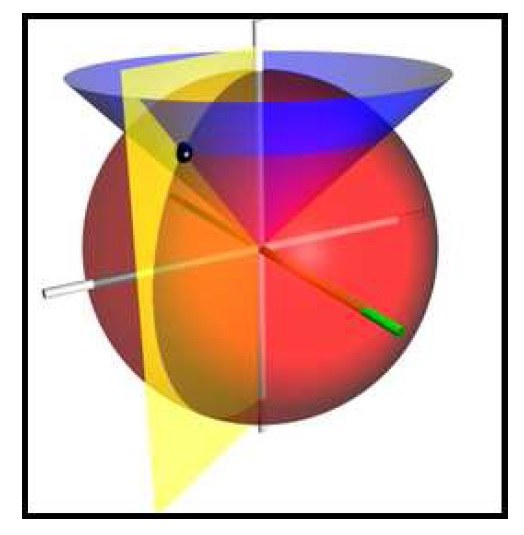### ACM 20150 Vector Integral and Differential CalculusI do not currently teach this module. The materials on this page are outdated and are left here for archival purposes only.

Description: This module introduces the fundamental concepts and methods in the differentiation and integration of vector and scalar fields, up to and including Gauss's and Stokes's theorems.

Topics and techniques discussed include but are not limited to the following list:

• Fundamentals Vectors and scalars: the dot and cross products, the geometry of lines and planes
• Taylor's theorem in several dimensions
• Curves in three-dimensional space: Differentiation of curves, the tangent vector
• Partial derivatives and vector fields: Introduction to partial derivatives, scalar and (Cartesian) vector fields, the operators div, grad, and curl in the Cartesian framework, applications of vector differentiation in electromagnetism and fluid mechanics
• Mutli-variate integration Area and volume as integrals, integrals of vector and scalar fields
• Stokes's and Gauss's theorems (statement and proof), Consequences of Stokes's and Gauss's theorems Green's theorems, the connection between vector fields that are derivable from a potential and irrotational vector fields
• Curvilinear coordinate systems: Basic concepts, the metric tensor, scale factors, div, grad, and curl in a general orthogonal curvilinear system, special curvilinear systems including spherical and cylindrical polar coordinates
• Further topics: These may include an introduction to differential forms, exact and inexact differential forms, advanced integration such as integrating the Gaussian function using polar coordinates, the gamma function, the volume of a four-ball by appropriate coordinate parameterization, the volume of a ball in an arbitrary (finite) number of dimensions using the gamma function

Learning Outcomes: On completion of this module students should be able to:

1. Write down parametric equations for lines and planes, and perform standard calculations based on these equations (e.g. points/lines of intersection, condition for lines to be skew)
2. Compute Taylor expansions for functions of one and several variables
3. Differentiate scalar and vector fields expressed in a Cartesian framework
4. Perform operations involving div, grad, and curl
5. Perform line, surface, and volume integrals. The geometric objects involved in the integrals may be lines, arbitrary curves, simple surfaces, and simple volumes, e.g. cubes, spheres, cylinders, and pyramids
6. State precisely and prove Gauss's and Stokes's theorems
7. Derive corollaries of these theorems, including Green's theorems and the necessary and sufficient condition for a vector field to be derivable from a potential
8. Compute the scale factors for arbitrary orthogonal curvilinear coordinate systems
9. Apply the formulas for div, grad, and curl in arbitrary orthogonal curvilinear coordinate systems

Course documents:

Recommended movies: